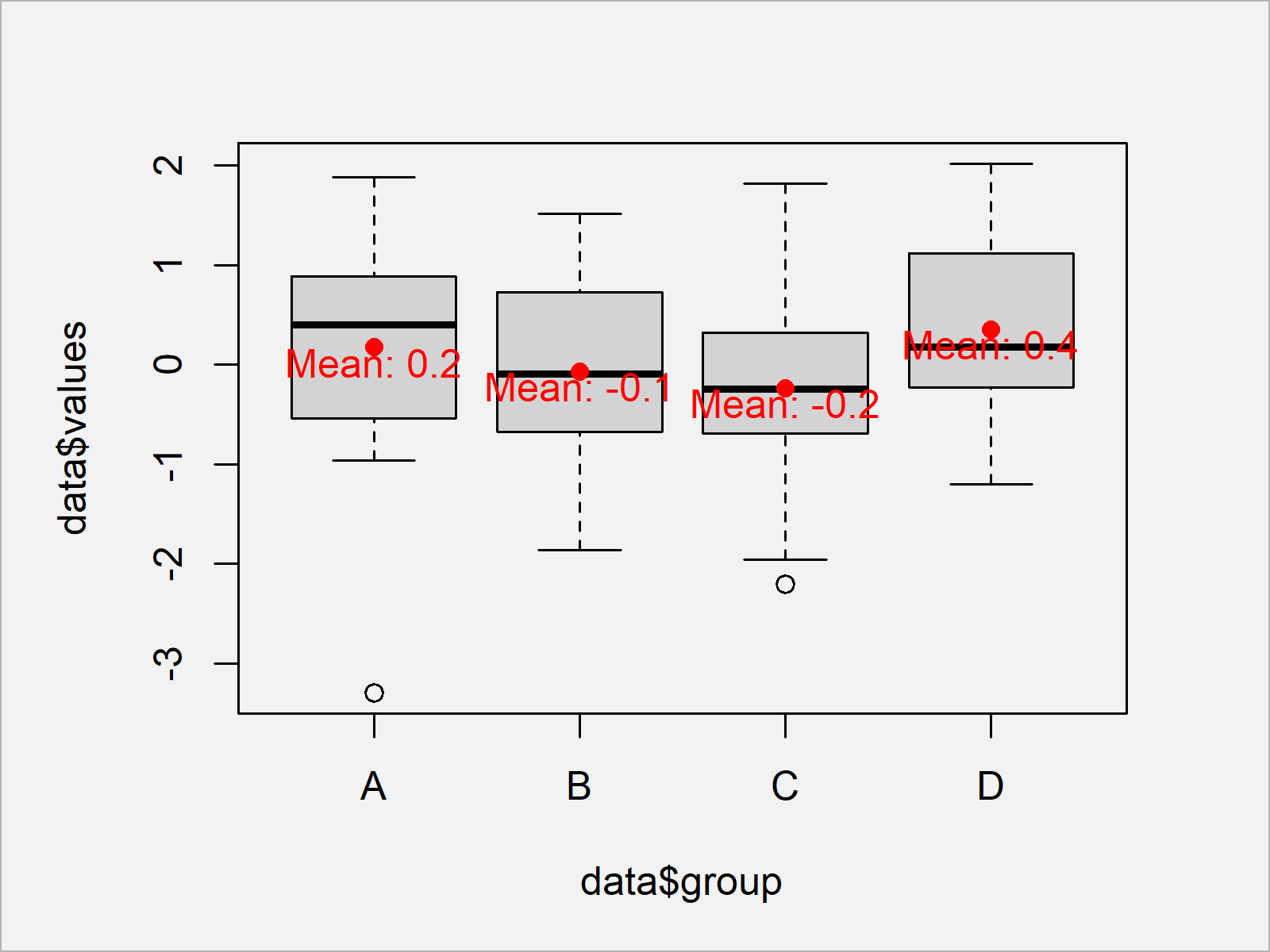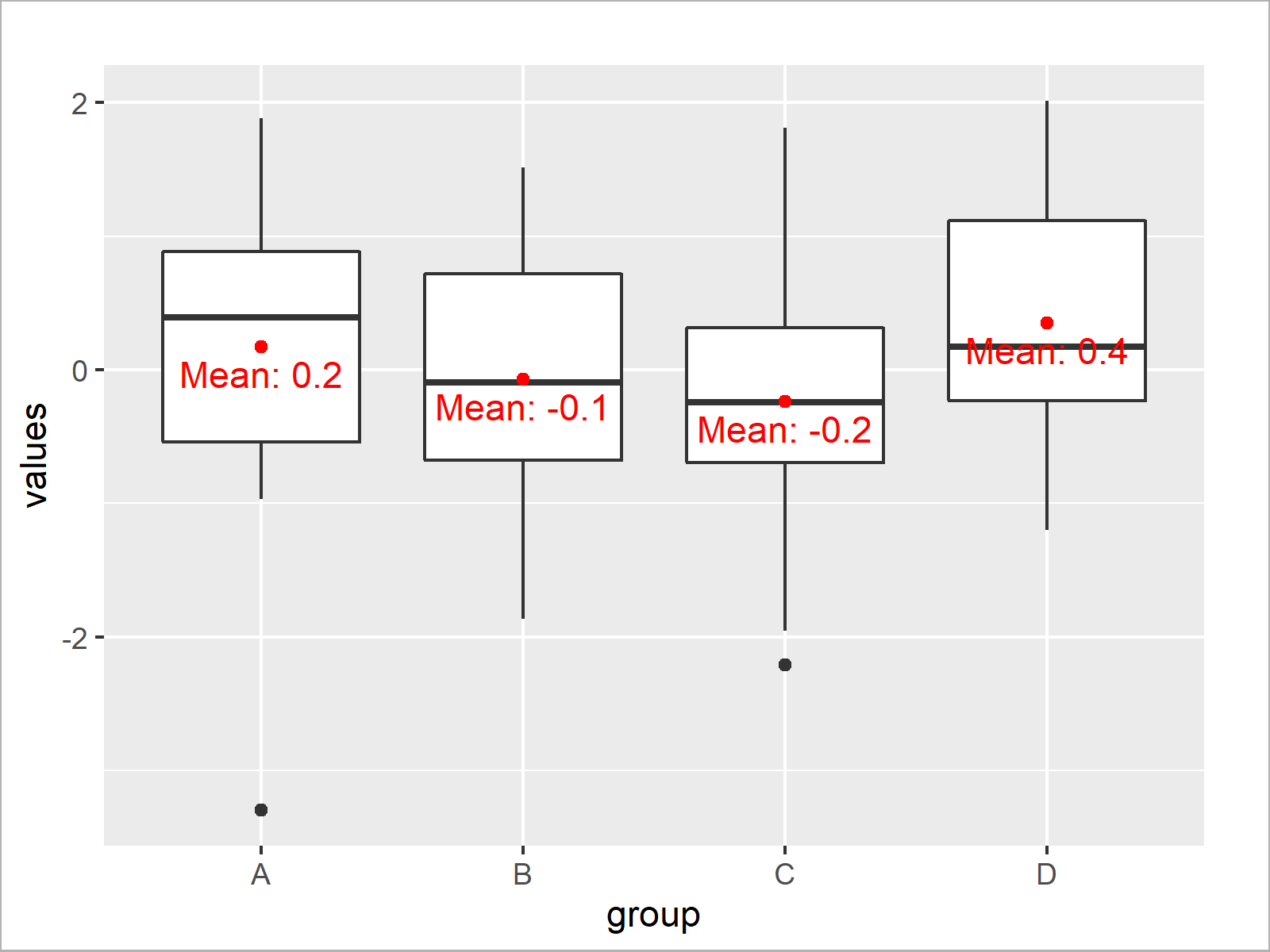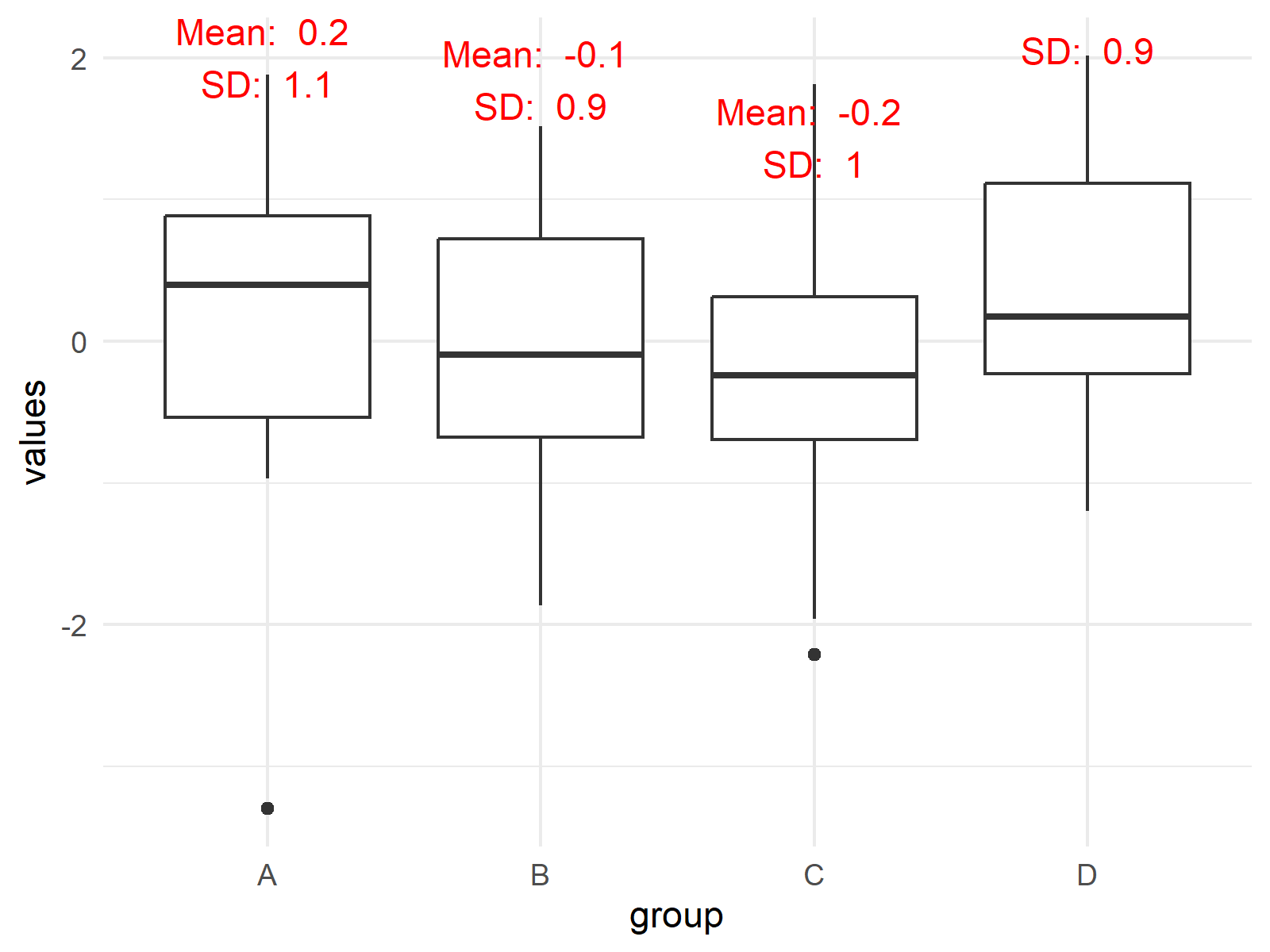# Draw Boxplot with Means in R (2 Examples)

In this R tutorial you’ll learn how to draw a box-whisker-plot with mean values.

The table of content is structured as follows:

Let’s dive into it.

## Creation of Exemplifying Data

Have a look at the following example data:

```set.seed(2967358)                                          # Create example data
data <- data.frame(values = rnorm(100),
group = LETTERS[1:4])
#       values group
# 1  0.5949582     A
# 2  0.9930561     B
# 3 -0.0228722     C
# 4  0.1745861     D
# 5  0.9405201     A
# 6 -1.1648994     B```

The previous output of the RStudio console visualizes that our example data has two columns. The variable values contains numeric data and the variable group consists of a group indicator.

## Example 1: Drawing Boxplot with Mean Values Using Base R

In Example 1, I’ll explain how to draw a boxplot with means using the basic features of the R programming language.

First, we have to apply the aggregate function to calculate mean values by group:

```data_means <- aggregate(data\$values,                       # Means by group
list(data\$group),
mean)
data_means                                                 # Print means by group
#   Group.1           x
# 1       A  0.17191346
# 2       B -0.06744831
# 3       C -0.23547290
# 4       D  0.35031848```

Now, we can apply the boxplot, points, and text functions to draw a boxplot with mean values in Base R:

```boxplot(data\$values ~ data\$group)                          # Draw boxplot in Base R
points(x = 1:nrow(data_means),                             # Add points to plot
y = data_means\$x,
col = "red",
pch = 16)
text(x = 1:nrow(data_means),                               # Add text to plot
y = data_means\$x - 0.15,
labels = paste("Mean:", round(data_means\$x, 1)),
col = "red")```Figure 1 shows the output of the previous R code – A box-and-whisker plot with mean values and text.

## Example 2: Drawing Boxplot with Mean Values Using ggplot2 Package

In Example 2, I’ll illustrate how to use the functions of the ggplot2 package to add mean values to a boxplot in R.

We first need to install and load the ggplot2 package, if we want to use the corresponding functions:

```install.packages("ggplot2")                                # Install & load ggplot2 package
library("ggplot2")```

Now, we can use the geom_boxplot and stat_summary functions to draw our boxplots with means:

```ggplot(data, aes(x = group, y = values)) +                 # Draw ggplot2 boxplot
geom_boxplot() +
stat_summary(fun = mean, geom = "point", col = "red") +  # Add points to plot
stat_summary(fun = mean, geom = "text", col = "red",     # Add text to plot
vjust = 1.5, aes(label = paste("Mean:", round(..y.., digits = 1))))```As shown in Figure 2, we have created a ggplot2 boxplot with mean values with the previously shown R code.

## Video & Further Resources

I have recently released a video on my YouTube channel, which explains the R codes of the present article. You can find the video below.

Furthermore, you may want to have a look at the other tutorials on this website. Some related articles about boxplots and other graphics in R can be found below:

On this page you learned how to create a boxplot with means in R. In case you have additional questions, don’t hesitate to let me know in the comments. Furthermore, don’t forget to subscribe to my email newsletter in order to get updates on the newest tutorials.

Subscribe to the Statistics Globe Newsletter

•September 20, 2021 3:24 am

Joachim:
Thank you very much for your explanation. With it I could draw boxplots (vertical and horizontal) with the mean value indicated (for one group) and with base R.

•September 20, 2021 6:19 am

Thank you very much for the kind comment Isaías! Great to hear that the tutorial helped you to create some nice graphics 🙂

Regards

Joachim

•Sofia
December 14, 2022 2:42 pm

Excellent explantation, I’ve been wandering a whole week trying to find the easiest way to draw mean and median in boxplots with ggplot2. Thank you !

Sofia

•December 15, 2022 9:50 am

You are welcome, Sofia! Have a good one 🙂

•Anni
June 9, 2023 10:34 am

One question, how can I have mean + standard deviation values shown above the box plots using ggplot 2? It would be visually more clear if the numbers are not inside the box plots. Thank you!

•June 12, 2023 8:48 am

Hello Anni,

Would something like the following help?

```# Calculate summary statistics
library(dplyr)
summ_stats <- data %>%
group_by(group) %>%
summarise(mean=mean(values), sd=sd(values), Q3=quantile(values, 0.75),  .groups = 'drop')

# Create the ggplot
library(ggplot2)

p <- ggplot(data, aes(x=group, y=values)) +
geom_boxplot() +
geom_text(data=summ_stats, aes(y=Q3, label=paste("Mean: ", round(mean, 1), "\nSD: ", round(sd, 1))), vjust=-1.5, color = "red") +
theme_minimal()

print(p)```